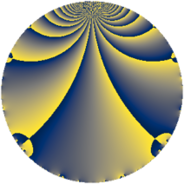# Properties

 Label 322.2.aLevel $322$ Weight $2$ Character orbit 322.a Rep. character $\chi_{322}(1,\cdot)$ Character field $\Q$ Dimension $11$ Newform subspaces $7$ Sturm bound $96$ Trace bound $3$

# Related objects

## Defining parameters

 Level: $$N$$ $$=$$ $$322 = 2 \cdot 7 \cdot 23$$ Weight: $$k$$ $$=$$ $$2$$ Character orbit: $$[\chi]$$ $$=$$ 322.a (trivial) Character field: $$\Q$$ Newform subspaces: $$7$$ Sturm bound: $$96$$ Trace bound: $$3$$ Distinguishing $$T_p$$: $$3$$, $$5$$

## Dimensions

The following table gives the dimensions of various subspaces of $$M_{2}(\Gamma_0(322))$$.

Total New Old
Modular forms 52 11 41
Cusp forms 45 11 34
Eisenstein series 7 0 7

The following table gives the dimensions of the cuspidal new subspaces with specified eigenvalues for the Atkin-Lehner operators and the Fricke involution.

$$2$$$$7$$$$23$$FrickeDim
$$+$$$$+$$$$+$$$+$$$2$$
$$+$$$$-$$$$+$$$-$$$1$$
$$+$$$$-$$$$-$$$+$$$1$$
$$-$$$$+$$$$+$$$-$$$3$$
$$-$$$$+$$$$-$$$+$$$1$$
$$-$$$$-$$$$-$$$-$$$3$$
Plus space$$+$$$$4$$
Minus space$$-$$$$7$$

## Trace form

 $$11 q + 3 q^{2} + 11 q^{4} - 2 q^{5} - q^{7} + 3 q^{8} + 15 q^{9} + O(q^{10})$$ $$11 q + 3 q^{2} + 11 q^{4} - 2 q^{5} - q^{7} + 3 q^{8} + 15 q^{9} + 6 q^{10} - 2 q^{13} - q^{14} - 16 q^{15} + 11 q^{16} - 10 q^{17} + 7 q^{18} - 8 q^{19} - 2 q^{20} + 4 q^{21} - q^{23} - 3 q^{25} - 2 q^{26} - 24 q^{27} - q^{28} + 2 q^{29} - 16 q^{31} + 3 q^{32} + 14 q^{34} - 2 q^{35} + 15 q^{36} - 10 q^{37} + 8 q^{38} - 40 q^{39} + 6 q^{40} - 18 q^{41} - 4 q^{42} - 8 q^{43} + 6 q^{45} + 3 q^{46} + 8 q^{47} + 11 q^{49} + 5 q^{50} + 32 q^{51} - 2 q^{52} - 18 q^{53} + 24 q^{54} - 8 q^{55} - q^{56} - 14 q^{58} - 16 q^{60} + 14 q^{61} - 16 q^{62} - 13 q^{63} + 11 q^{64} + 36 q^{65} - 16 q^{66} - 10 q^{68} - 4 q^{69} - 2 q^{70} - 24 q^{71} + 7 q^{72} - 10 q^{73} - 10 q^{74} - 24 q^{75} - 8 q^{76} + 12 q^{77} - 8 q^{78} - 32 q^{79} - 2 q^{80} + 3 q^{81} + 30 q^{82} + 16 q^{83} + 4 q^{84} + 44 q^{85} + 24 q^{86} + 24 q^{87} + 6 q^{89} - 34 q^{90} + 10 q^{91} - q^{92} + 24 q^{93} + 8 q^{94} - 8 q^{95} + 6 q^{97} + 3 q^{98} + O(q^{100})$$

## Decomposition of $$S_{2}^{\mathrm{new}}(\Gamma_0(322))$$ into newform subspaces

Label Dim $A$ Field CM Traces A-L signs $q$-expansion
$a_{2}$ $a_{3}$ $a_{5}$ $a_{7}$ 2 7 23
322.2.a.a $1$ $2.571$ $$\Q$$ None $$-1$$ $$0$$ $$-2$$ $$1$$ $+$ $-$ $-$ $$q-q^{2}+q^{4}-2q^{5}+q^{7}-q^{8}-3q^{9}+\cdots$$
322.2.a.b $1$ $2.571$ $$\Q$$ None $$-1$$ $$2$$ $$0$$ $$1$$ $+$ $-$ $+$ $$q-q^{2}+2q^{3}+q^{4}-2q^{6}+q^{7}-q^{8}+\cdots$$
322.2.a.c $1$ $2.571$ $$\Q$$ None $$1$$ $$-2$$ $$-2$$ $$-1$$ $-$ $+$ $-$ $$q+q^{2}-2q^{3}+q^{4}-2q^{5}-2q^{6}-q^{7}+\cdots$$
322.2.a.d $1$ $2.571$ $$\Q$$ None $$1$$ $$2$$ $$-2$$ $$1$$ $-$ $-$ $-$ $$q+q^{2}+2q^{3}+q^{4}-2q^{5}+2q^{6}+q^{7}+\cdots$$
322.2.a.e $2$ $2.571$ $$\Q(\sqrt{5})$$ None $$-2$$ $$-2$$ $$-2$$ $$-2$$ $+$ $+$ $+$ $$q-q^{2}+(-1-\beta )q^{3}+q^{4}+(-1+\beta )q^{5}+\cdots$$
322.2.a.f $2$ $2.571$ $$\Q(\sqrt{3})$$ None $$2$$ $$-2$$ $$2$$ $$2$$ $-$ $-$ $-$ $$q+q^{2}+(-1+\beta )q^{3}+q^{4}+(1+\beta )q^{5}+\cdots$$
322.2.a.g $3$ $2.571$ 3.3.316.1 None $$3$$ $$2$$ $$4$$ $$-3$$ $-$ $+$ $+$ $$q+q^{2}+(1+\beta _{2})q^{3}+q^{4}+(1-\beta _{2})q^{5}+\cdots$$

## Decomposition of $$S_{2}^{\mathrm{old}}(\Gamma_0(322))$$ into lower level spaces

$$S_{2}^{\mathrm{old}}(\Gamma_0(322)) \cong$$ $$S_{2}^{\mathrm{new}}(\Gamma_0(14))$$$$^{\oplus 2}$$$$\oplus$$$$S_{2}^{\mathrm{new}}(\Gamma_0(23))$$$$^{\oplus 4}$$$$\oplus$$$$S_{2}^{\mathrm{new}}(\Gamma_0(46))$$$$^{\oplus 2}$$$$\oplus$$$$S_{2}^{\mathrm{new}}(\Gamma_0(161))$$$$^{\oplus 2}$$